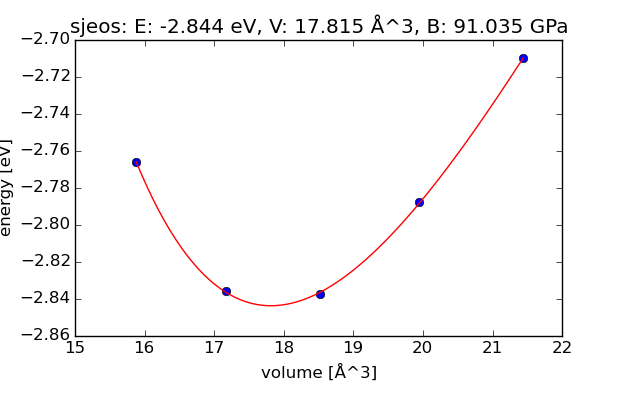# GPAW Example

GPAW is a density-functional theory (DFT) Python code based on the projector-augmented wave (PAW) method and the atomic simulation environment (ASE). The wave functions can be described with plane-waves, real-space uniform grids, multigrid methods and the finite-difference approximation, atom-centered basis-functions.

Given below is an example of GPAW simulation on Rescale using MPI parallelization.Post processing visualization of results

 Simulation Code GPAW 0.10.0 or newer (1.1.0 in linked example) Analysis Type Solid-state physics Description GPAW example using MPI: equation of state of FCC silver Suggested Hardware Onyx / 2 cores Command `mpirun -np 1 gpaw-python eos1-gpaw.py` → `gpaw-python eos2-gpaw.py` Estimated Run Time 2 minutes Contributor Marcin Dulak